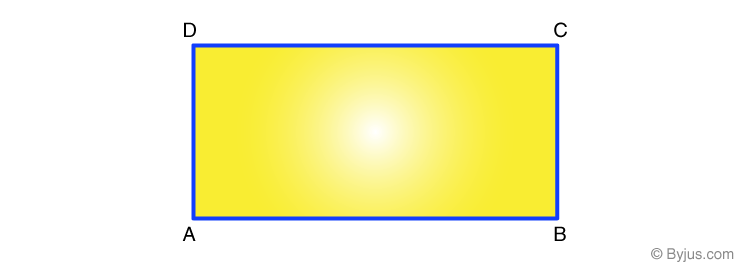# Properties of Rectangle

A rectangle is a two-dimensional plane figure with four sides. A rectangle is a four-sided polygon in which the opposite sides are parallel and equal to each other. It is one of the types of quadrilaterals in which all four angles are right angles or equal to 90 degrees. The rectangle is a special type of parallelogram with all its angles equal. A rectangle with four equal sides is known as a square. Now let us learn the properties of rectangle in this article.## Rectangle Properties

The fundamental properties of rectangles are:

• A rectangle is a quadrilateral
• The opposite sides are parallel and equal to each other
• Each interior angle is equal to 90 degrees
• The sum of all the interior angles is equal to 360 degrees
• The diagonals bisect each other
• Both the diagonals have the same length
• A rectangle with side lengths a and b has the perimeter as 2a+2b units
• A rectangle with side lengths a and b has the area as: ab sin 90 = ab square units
• A diagonal of a rectangle is the diameter of its circumcircle
• If a and b are the sides of a rectangle, then the length of each diagonal is:
$$\begin{array}{l}d = \sqrt{a^{2}+b^{2}}\end{array}$$
• The diagonals bisect each other at different angles. One is acute, and another one is an obtuse angle
• If the two diagonals bisect each other at right angles, then the rectangle is known as a square
• A cylinder is obtained when the rectangle is rotated along the line joining the midpoint of the longer parallel sides. In this case, the height of the cylinder is equal to the width of a rectangle. Also, the cylinder diameter is equivalent to the length of a rectangle
• A cylinder is obtained when the rectangle is rotated along the line joining the midpoint of the shorter parallel sides. In this case, the height of the cylinder is equal to the length of a rectangle. Similarly, the cylinder diameter is equivalent to the width of a rectangle

### Video Lesson## Solved Examples

Question 1:

Find the perimeter of a rectangle whose sides are 10 cm and 5 cm, respectively.

Solution:

Given:

a = 10 cm

b = 5 cm

According to the properties of a rectangle, the perimeter of a rectangle is:

Perimeter, P = 2a +2b units

P = 2 (a+b) units

Now, substitute the values

P = 2(10+5)

P = 2(15)

P = 30 cm

Therefore, the perimeter of a rectangle is 30 cm.

Question 2:

What is the area and length of the diagonal of a rectangle with adjacent side lengths of 24 cm and 7 cm?

Solution:

Let the measures of adjacent sides of a rectangle be:

a = 24 cm

b = 7 cm

As we know,

Area of a rectangle = ab

= 24 × 7

= 168 cm2

Therefore, the area of a rectangle is 168 cm2.

Diagonal of the rectangle = √(a2 + b2)

= √(242 + 72)

= √(576 + 49)

= √625

= 25 cm

Hence, the diagonal of the rectangle is 25 cm.

### Practice Problems

1. What is the breadth and perimeter of the rectangle if its area is 96 square units and the length is 12 units?
2. The diagonal of a rectangle has a length of 100 cm, and its length is twice its width. Find the area of this triangle.
3. Find the area and perimeter of a rectangle whose adjacent sides measure 19 m and 12 m.

Stay tuned with BYJU’S – The Learning App for the properties of different geometric shapes and explore videos.

## Frequently Asked Questions – FAQs

Q1

### What is the sum of all interior angles of a rectangle?

The sum of all interior angles of a rectangle is 360 degrees.
Q2

### Are the diagonals of a rectangle equal?

Yes, the diagonals of a rectangle are equal.
Q3

### What is the length property of a rectangle?

The length property of a rectangle states that the opposite sides of a rectangle are parallel and equal in length.
Q4

### Write the properties of diagonals of a rectangle.

In a rectangle,
Diagonals are equal in length
Diagonals bisect each other
If two diagonals bisect each other at different angles, then one is an acute angle, and another one is an obtuse angle
Quiz on Properties of Rectangle